Want to share your content on R-bloggers? click here if you have a blog, or here if you don't.

I’m very pleased to announce survminer 0.2.4. It comes with many new features and minor changes.

Install survminer with:

`install.packages("survminer")`

To load the package, type this:

`library(survminer)`

## New features

• New function `surv_summary()` for creating data frame containing a nice summary of survival curves (#64).
• It’s possible now to facet the output of `ggsurvplot()` by one or more factors (#64).
• Now, `ggsurvplot()` can be used to plot cox model (#67).
• New functions added for determining and visualizing the optimal cutpoint of continuous variables for survival analyses:
• `surv_cutpoint()`: Determine the optimal cutpoint for each variable using ‘maxstat’. Methods defined for surv_cutpoint object are summary(), print() and plot().
• `surv_categorize()`: Divide each variable values based on the cutpoint returned by `surv_cutpoint()` (#41).
• New argument ‘ncensor.plot’ added to `ggsurvplot()`. A logical value. If TRUE, the number of censored subjects at time t is plotted. Default is FALSE (#18).

## Minor changes

• New argument ‘conf.int.style’ added in `ggsurvplot()` for changing the style of confidence interval bands.
• Now, `ggsurvplot()` plots a stepped confidence interval when conf.int = TRUE (#65).
• `ggsurvplot()` updated for compatibility with the future version of ggplot2 (v2.2.0) (#68)
• ylab is now automatically adapted according to the value of the argument `fun`. For example, if fun = “event”, then ylab will be “Cumulative event”.
• In `ggsurvplot()`, linetypes can now be adjusted by variables used to fit survival curves (#46)
• In `ggsurvplot()`, the argument risk.table can be either a logical value (TRUE|FALSE) or a string (“absolute”, “percentage”). If risk.table = “absolute”, `ggsurvplot()` displays the absolute number of subjects at risk. If risk.table = “percentage”, the percentage at risk is displayed. Use “abs_pct” to show both the absolute number and the percentage of subjects at risk. (#70).
• New argument surv.median.line in `ggsurvplot()`: character vector for drawing a horizontal/vertical line at median (50%) survival. Allowed values include one of c(“none”, “hv”, “h”, “v”). v: vertical, h:horizontal (#61).
• Now, the default theme of ggcoxdiagnostics() is ggplot2::theme_bw().

## Bug fixes

It also includes numerous bug fixes as described in the release notes: v0.2.3 and v0.2.4

## Summary of survival curves

Compared to the default summary() function, the surv_summary() function [in survminer] creates a data frame containing a nice summary from survfit results.

```# Fit survival curves
require("survival")
fit <- survfit(Surv(time, status) ~ sex, data = lung)

# Summarize
library("survminer")
res.sum <- surv_summary(fit)
##   time n.risk n.event n.censor      surv    std.err     upper     lower
## 1   11    138       3        0 0.9782609 0.01268978 1.0000000 0.9542301
## 2   12    135       1        0 0.9710145 0.01470747 0.9994124 0.9434235
## 3   13    134       2        0 0.9565217 0.01814885 0.9911586 0.9230952
## 4   15    132       1        0 0.9492754 0.01967768 0.9866017 0.9133612
## 5   26    131       1        0 0.9420290 0.02111708 0.9818365 0.9038355
## 6   30    130       1        0 0.9347826 0.02248469 0.9768989 0.8944820
##   strata sex
## 1  sex=1   1
## 2  sex=1   1
## 3  sex=1   1
## 4  sex=1   1
## 5  sex=1   1
## 6  sex=1   1
# Information about the survival curves
attr(res.sum, "table")
##       records n.max n.start events   *rmean *se(rmean) median 0.95LCL
## sex=1     138   138     138    112 325.0663   22.59845    270     212
## sex=2      90    90      90     53 458.2757   33.78530    426     348
##       0.95UCL
## sex=1     310
## sex=2     550```

## Plot survival curves

```ggsurvplot(
fit,                     # survfit object with calculated statistics.
pval = TRUE,             # show p-value of log-rank test.
conf.int = TRUE,         # show confidence intervals for
# point estimaes of survival curves.
#conf.int.style = "step",  # customize style of confidence intervals
xlab = "Time in days",   # customize X axis label.
break.time.by = 200,     # break X axis in time intervals by 200.
ggtheme = theme_light(), # customize plot and risk table with a theme.
risk.table = "abs_pct",  # absolute number and percentage at risk.
risk.table.y.text.col = T,# colour risk table text annotations.
risk.table.y.text = FALSE,# show bars instead of names in text annotations
# in legend of risk table.
ncensor.plot = TRUE,      # plot the number of censored subjects at time t
surv.median.line = "hv",  # add the median survival pointer.
legend.labs =
c("Male", "Female"),    # change legend labels.
palette =
c("#E7B800", "#2E9FDF") # custom color palettes.
)```survminer

## Determine the optimal cutpoint for continuous variables

The survminer package determines the optimal cutpoint for one or multiple continuous variables at once, using the maximally selected rank statistics from the ‘maxstat’ R package. To learn more, read this: M. Kosiński. R-ADDICT November 2016. Determine optimal cutpoints for numerical variables in survival plots.

Here, we’ll use the myeloma data sets [in the survminer package]. It contains survival data and some gene expression data obtained from multiple myeloma patients.

```# 0. Load some data
data(myeloma)
##          molecular_group chr1q21_status treatment event  time   CCND1
## GSM50986      Cyclin D-1       3 copies       TT2     0 69.24  9908.4
## GSM50988      Cyclin D-2       2 copies       TT2     0 66.43 16698.8
## GSM50989           MMSET       2 copies       TT2     0 66.50   294.5
## GSM50990           MMSET       3 copies       TT2     1 42.67   241.9
## GSM50991             MAF                  TT2     0 65.00   472.6
## GSM50992    Hyperdiploid       2 copies       TT2     0 65.20   664.1
##          CRIM1 DEPDC1
## GSM50986 420.9  523.5
## GSM50988  52.0   21.1
## GSM50989 617.9  192.9
## GSM50990  11.9  184.7
## GSM50991  38.8  212.0
## GSM50992  16.9  341.6
# 1. Determine the optimal cutpoint of variables
res.cut <- surv_cutpoint(myeloma, time = "time", event = "event",
variables = c("DEPDC1", "WHSC1", "CRIM1"))

summary(res.cut)
##        cutpoint statistic
## DEPDC1    279.8  4.275452
## WHSC1    3205.6  3.361330
## CRIM1      82.3  1.968317
# 2. Plot cutpoint for DEPDC1
# palette = "npg" (nature publishing group), see ?ggpubr::ggpar
plot(res.cut, "DEPDC1", palette = "npg")
## \$DEPDC1```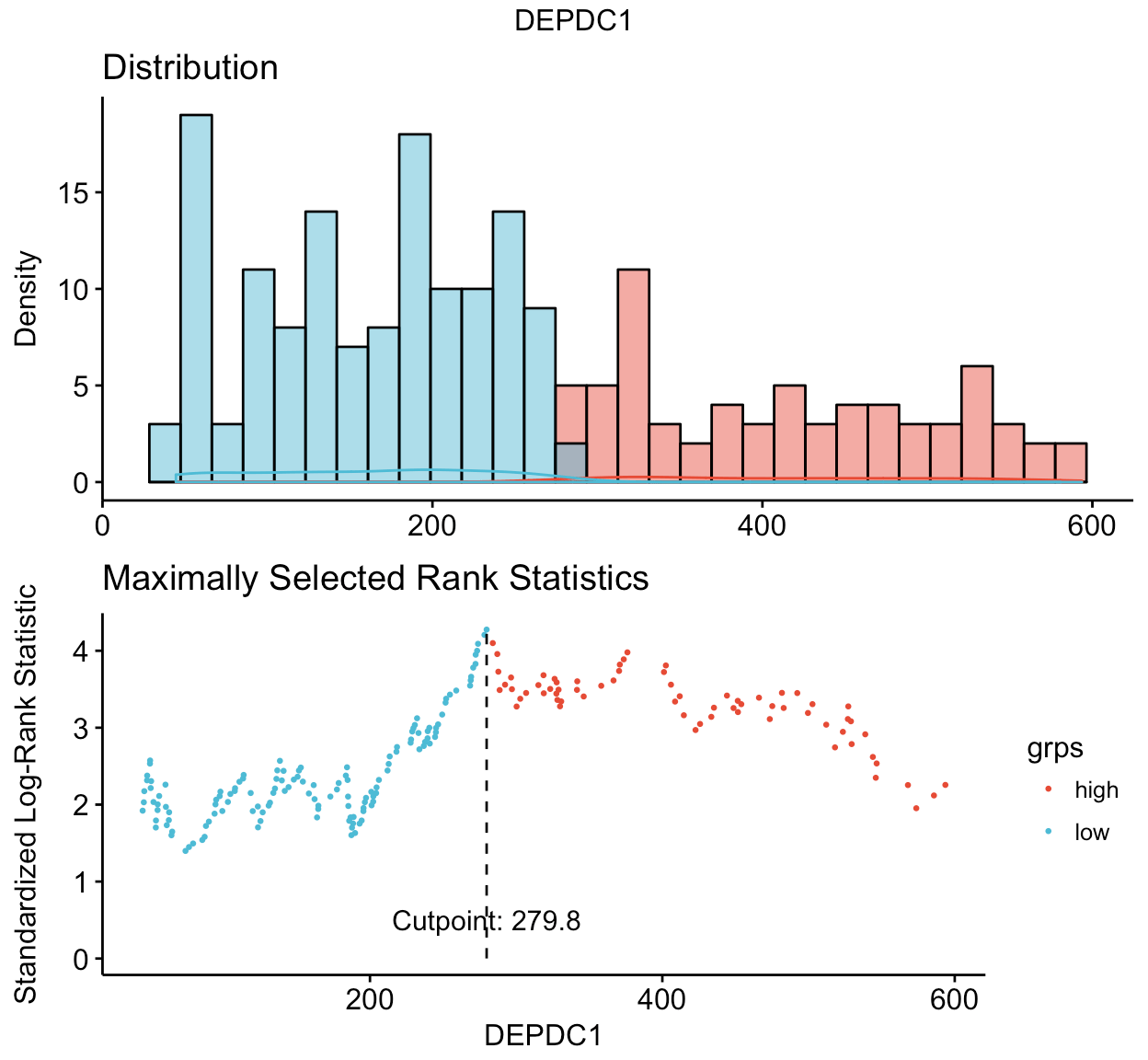survminer

```# 3. Categorize variables
res.cat <- surv_categorize(res.cut)
##           time event DEPDC1 WHSC1 CRIM1
## GSM50986 69.24     0   high   low  high
## GSM50988 66.43     0    low   low   low
## GSM50989 66.50     0    low  high  high
## GSM50990 42.67     1    low  high   low
## GSM50991 65.00     0    low   low   low
## GSM50992 65.20     0   high   low   low
# 4. Fit survival curves and visualize
library("survival")
fit <- survfit(Surv(time, event) ~DEPDC1, data = res.cat)
ggsurvplot(fit, risk.table = TRUE, conf.int = TRUE)```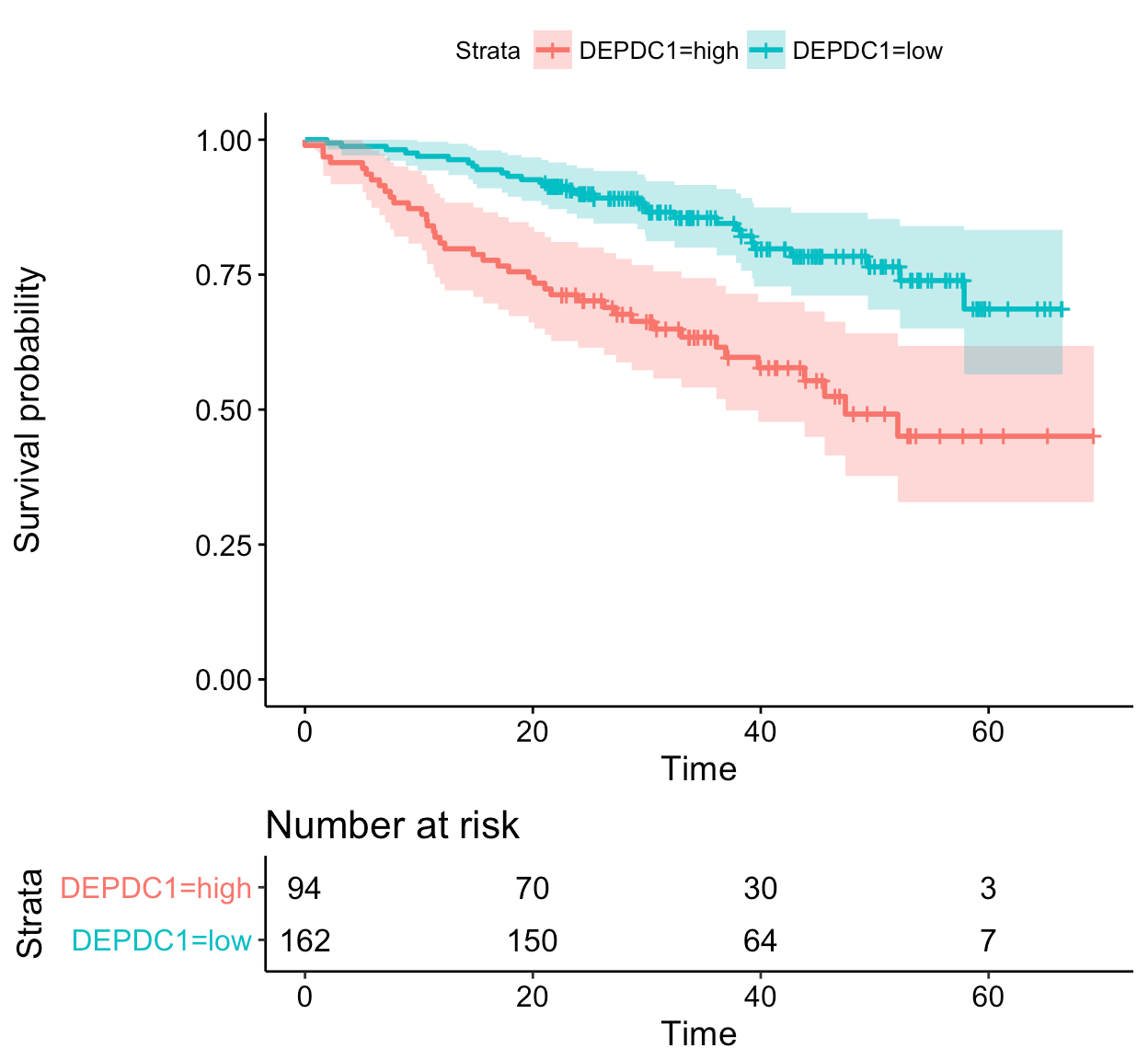survminer

## Facet the output of ggsurvplot()

In this section, we’ll compute survival curves using the combination of multiple factors. Next, we’ll factet the output of ggsurvplot() by a combination of factors

1. Fit (complex) survival curves using colon data sets
```require("survival")
fit2 <- survfit( Surv(time, status) ~ sex + rx + adhere,
data = colon )```
1. Visualize the output using survminer
```ggsurv <- ggsurvplot(fit2, fun = "event", conf.int = TRUE,
risk.table = TRUE, risk.table.col="strata",
ggtheme = theme_bw())

ggsurv```survminer

1. Faceting survival curves. The plot below shows survival curves by the sex variable faceted according to the values of rx & adhere.
```curv_facet <- ggsurv\$plot + facet_grid(rx ~ adhere)
curv_facet```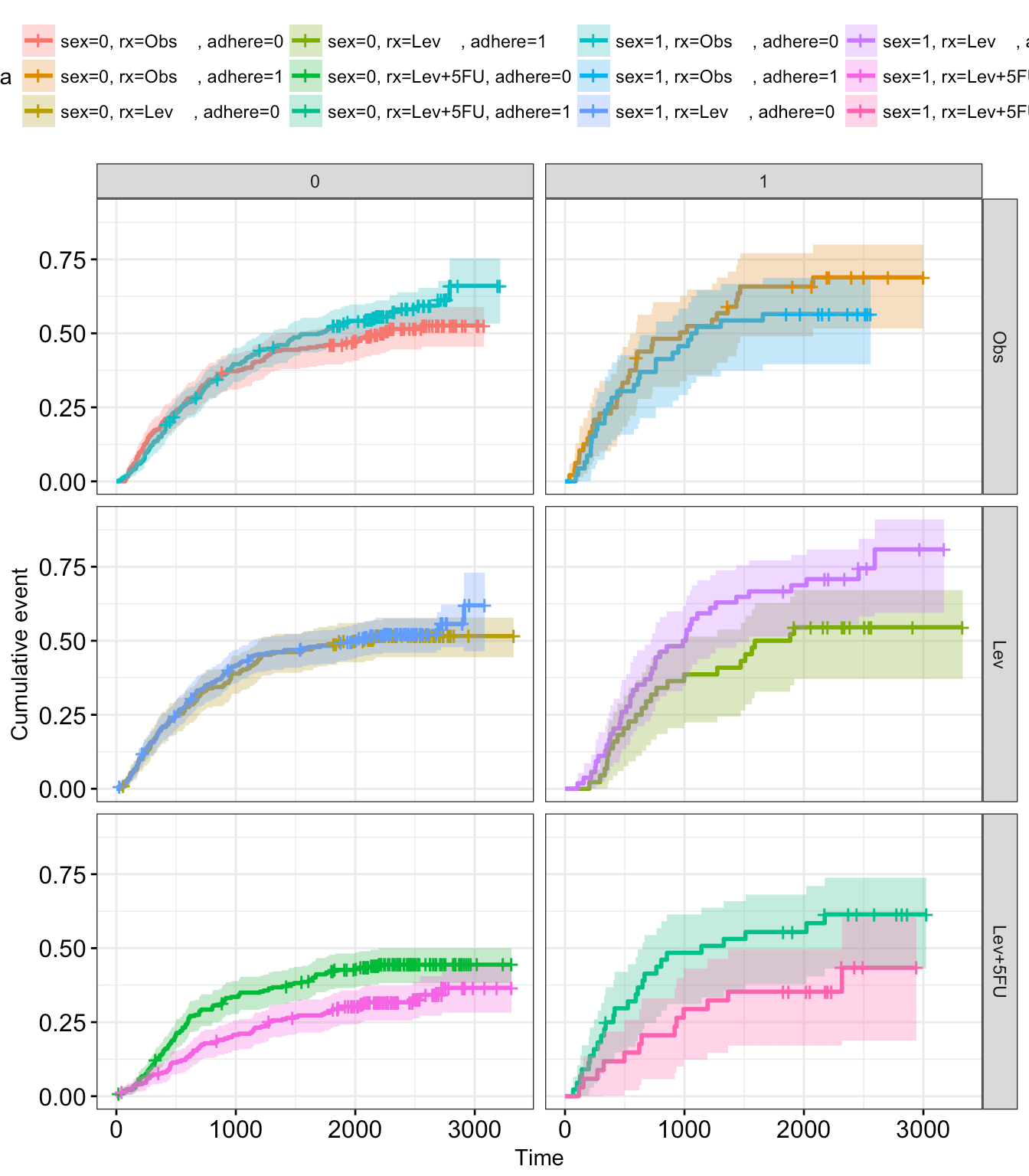survminer

1. Facetting risk tables: Generate risk table for each facet plot item
```ggsurv\$table + facet_grid(rx ~ adhere, scales = "free")+
theme(legend.position = "none")```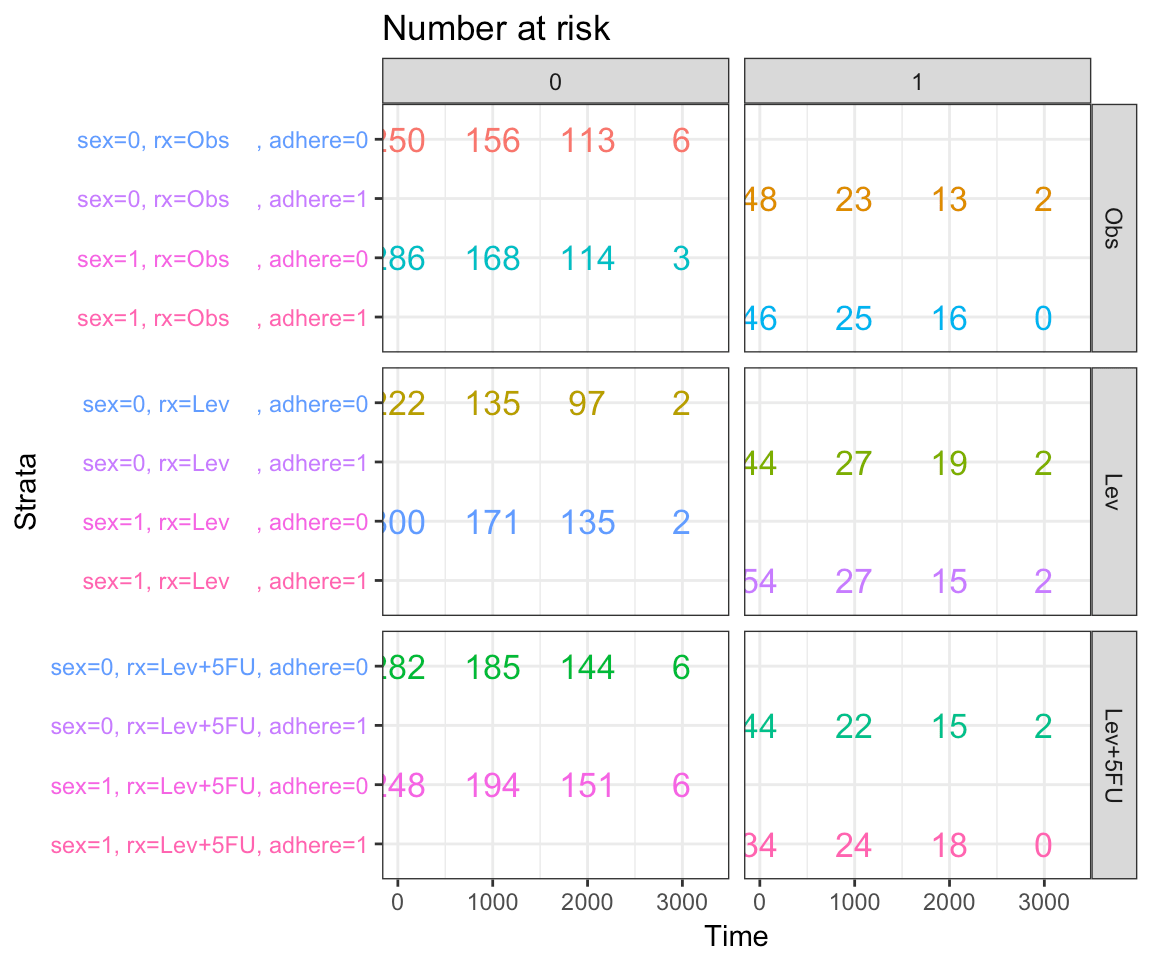survminer

1. Generate risk table for each facet columns
```tbl_facet <- ggsurv\$table + facet_grid(.~ adhere, scales = "free")
tbl_facet + theme(legend.position = "none")```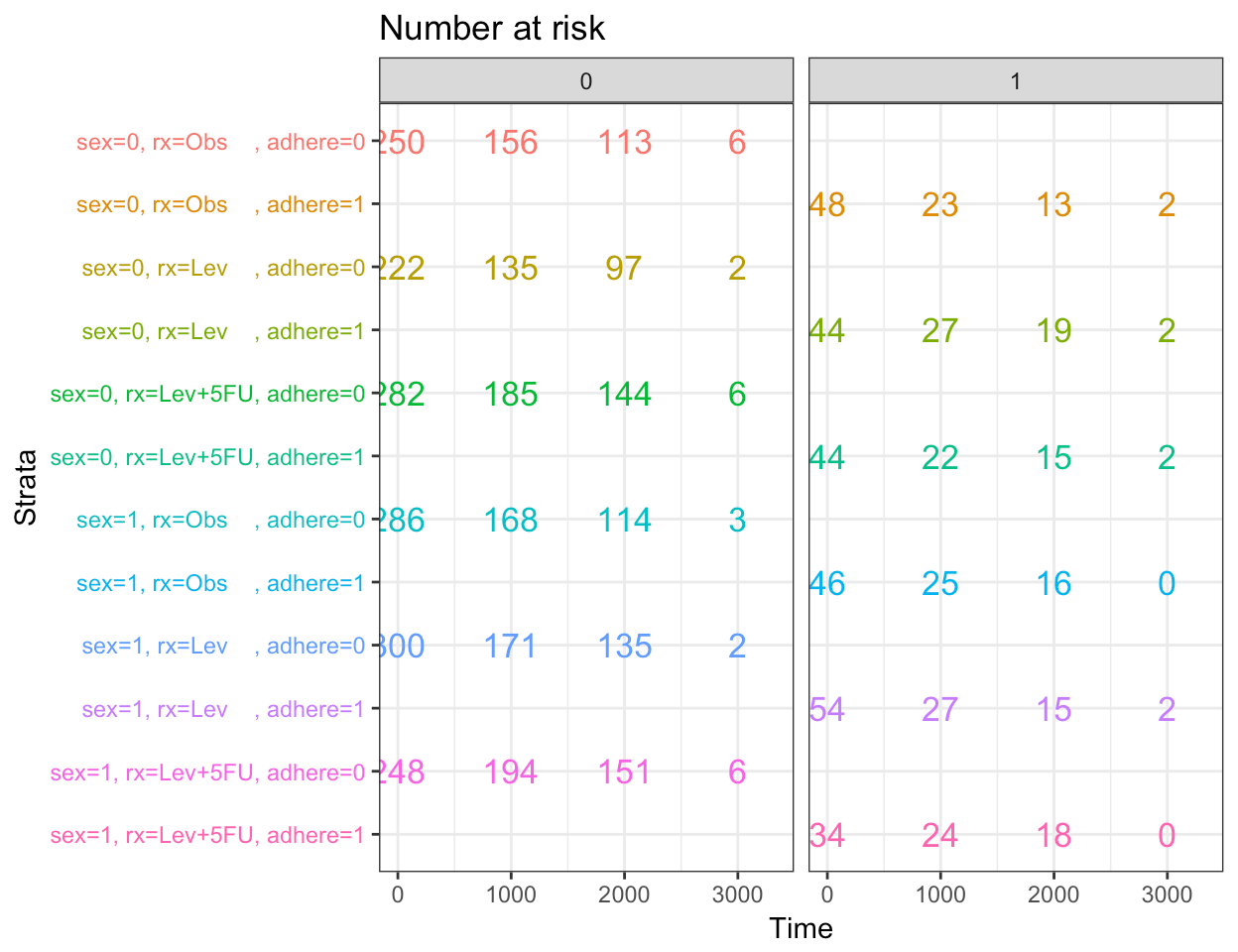survminer

```# Arrange faceted survival curves and risk tables
g2 <- ggplotGrob(curv_facet)
g3 <- ggplotGrob(tbl_facet)
min_ncol <- min(ncol(g2), ncol(g3))
g <- gridExtra::rbind.gtable(g2[, 1:min_ncol], g3[, 1:min_ncol], size="last")
g\$widths <- grid::unit.pmax(g2\$widths, g3\$widths)
grid::grid.newpage()
grid::grid.draw(g)```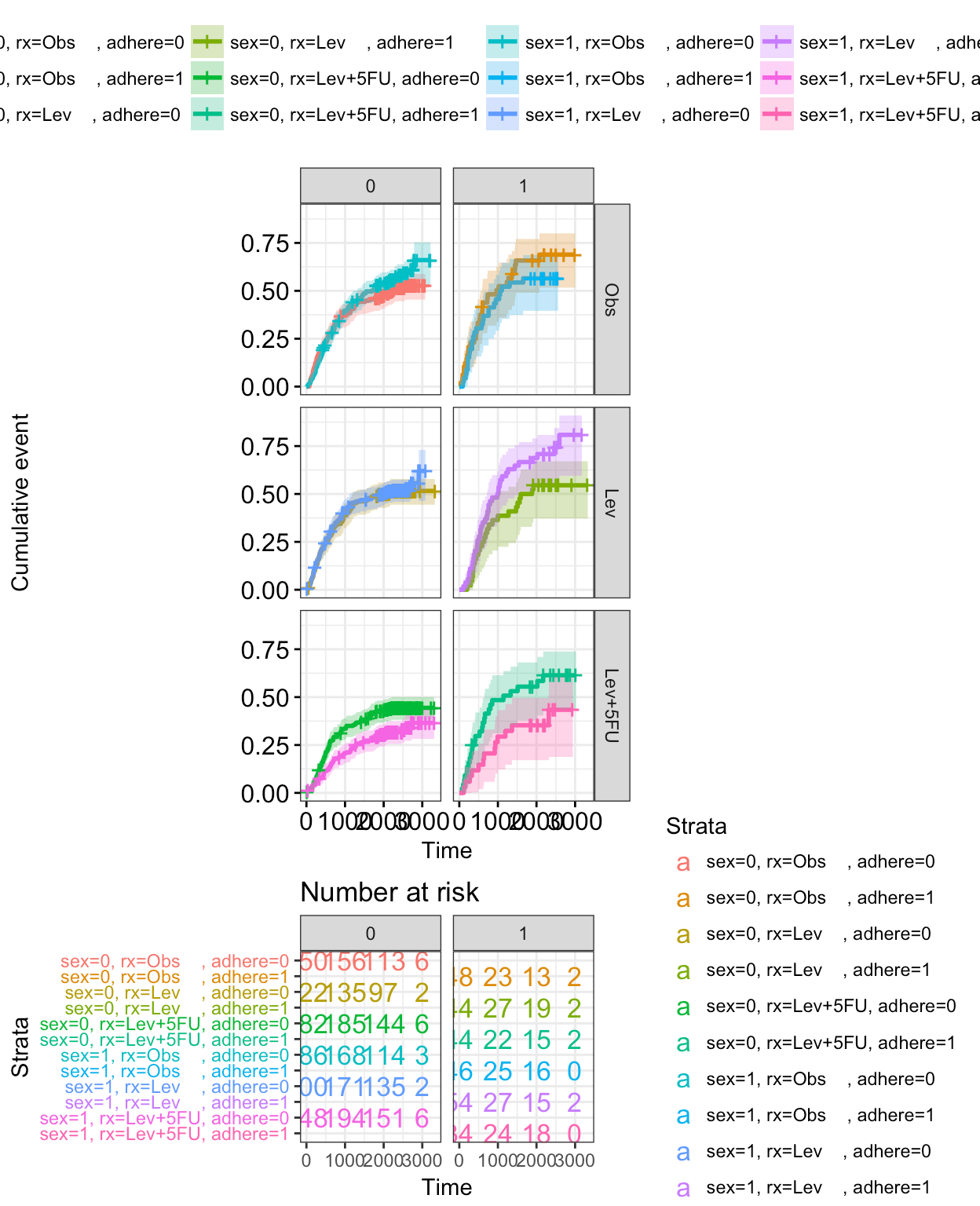survminer

## Infos

This analysis has been performed using R software (ver. 3.3.2).